Скачать презентацию University of Perugia Department of Economics Energy demand

57b634fb48785f353d9f8769632ef06d.ppt

• Количество слайдов: 26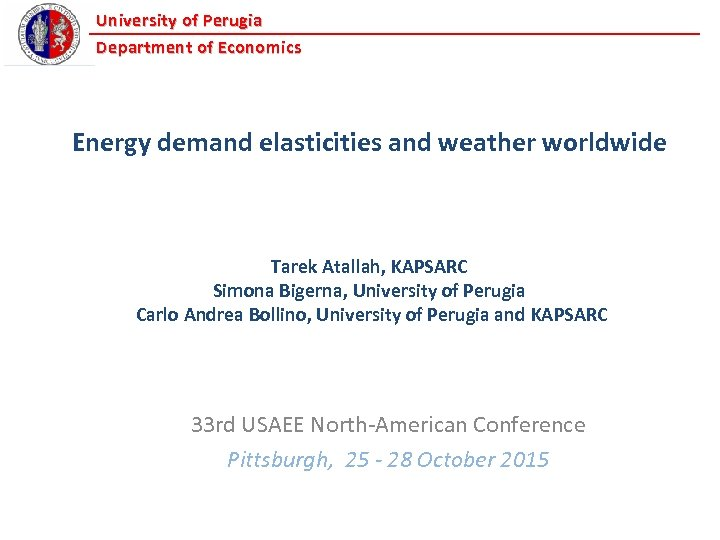University of Perugia Department of Economics Energy demand elasticities and weather worldwide Tarek Atallah, KAPSARC Simona Bigerna, University of Perugia Carlo Andrea Bollino, University of Perugia and KAPSARC 33 rd USAEE North-American Conference Pittsburgh, 25 - 28 October 2015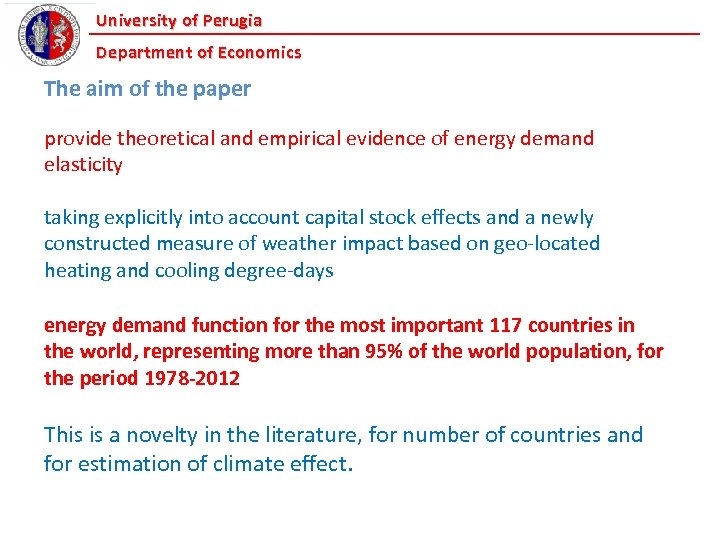University of Perugia Department of Economics The aim of the paper provide theoretical and empirical evidence of energy demand elasticity taking explicitly into account capital stock effects and a newly constructed measure of weather impact based on geo-located heating and cooling degree-days energy demand function for the most important 117 countries in the world, representing more than 95% of the world population, for the period 1978 -2012 This is a novelty in the literature, for number of countries and for estimation of climate effect.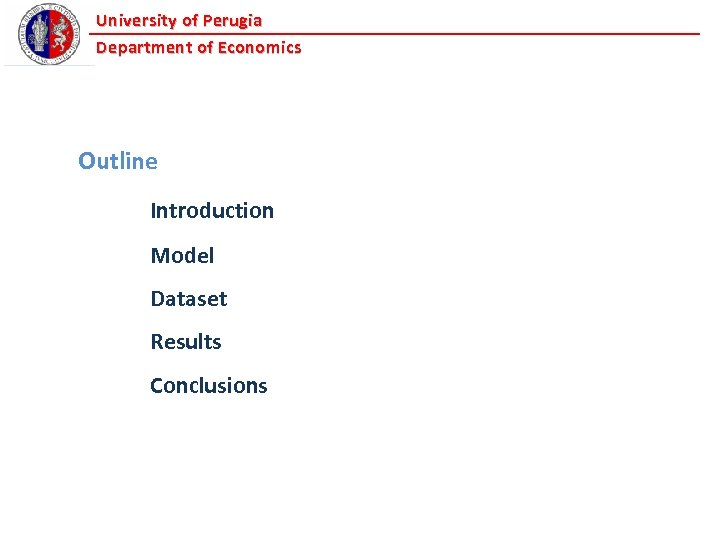University of Perugia Department of Economics Outline Introduction Model Dataset Results Conclusions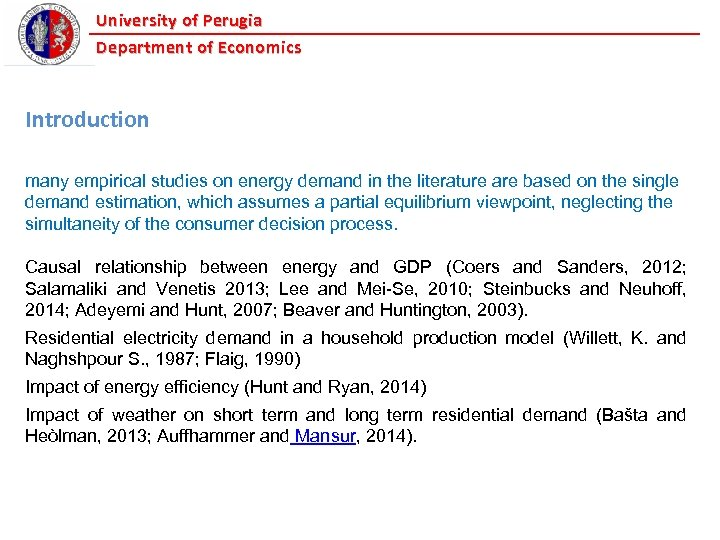University of Perugia Department of Economics Introduction many empirical studies on energy demand in the literature are based on the single demand estimation, which assumes a partial equilibrium viewpoint, neglecting the simultaneity of the consumer decision process. Causal relationship between energy and GDP (Coers and Sanders, 2012; Salamaliki and Venetis 2013; Lee and Mei-Se, 2010; Steinbucks and Neuhoff, 2014; Adeyemi and Hunt, 2007; Beaver and Huntington, 2003). Residential electricity demand in a household production model (Willett, K. and Naghshpour S. , 1987; Flaig, 1990) Impact of energy efficiency (Hunt and Ryan, 2014) Impact of weather on short term and long term residential demand (Bašta and Heòlman, 2013; Auffhammer and Mansur, 2014).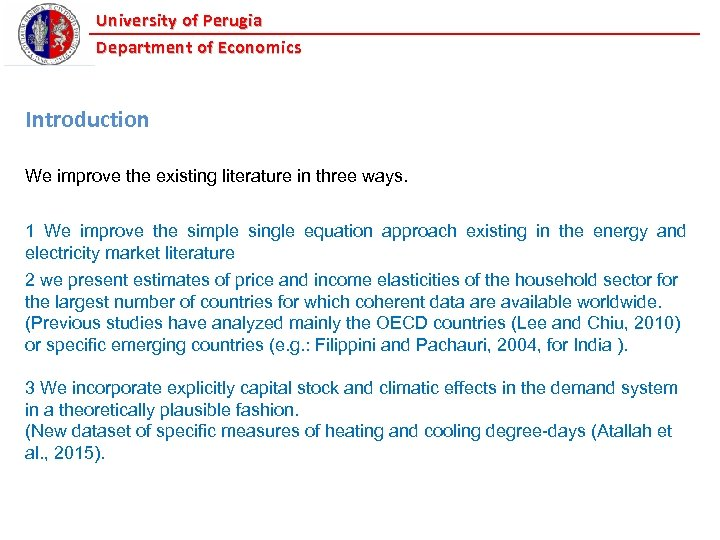University of Perugia Department of Economics Introduction We improve the existing literature in three ways. 1 We improve the simple single equation approach existing in the energy and electricity market literature 2 we present estimates of price and income elasticities of the household sector for the largest number of countries for which coherent data are available worldwide. (Previous studies have analyzed mainly the OECD countries (Lee and Chiu, 2010) or specific emerging countries (e. g. : Filippini and Pachauri, 2004, for India ). 3 We incorporate explicitly capital stock and climatic effects in the demand system in a theoretically plausible fashion. (New dataset of specific measures of heating and cooling degree-days (Atallah et al. , 2015).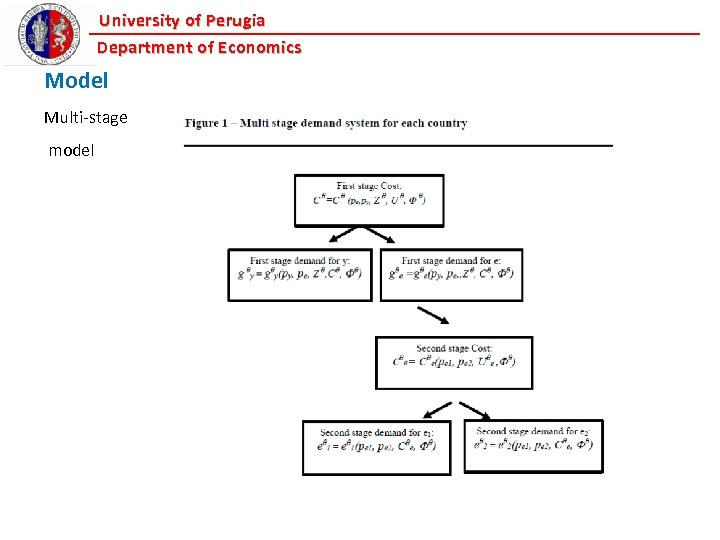University of Perugia Department of Economics Model Multi-stage model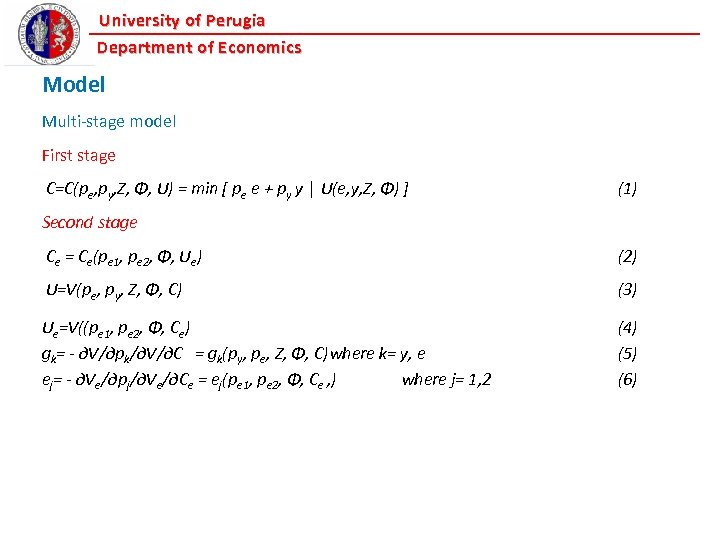University of Perugia Department of Economics Model Multi-stage model First stage C=C(pe, py, Z, Φ, U) = min [ pe e + py y │ U(e, y, Z, Φ) ] (1) Second stage Ce = Ce(pe 1, pe 2, Φ, Ue) (2) U=V(pe, py, Z, Φ, C) (3) Ue=V((pe 1, pe 2, Φ, Ce) gk= - ∂V/∂pk/∂V/∂C = gk(py, pe, Z, Φ, C)where k= y, e ej= - ∂Ve/∂pj/∂Ve/∂Ce = ej(pe 1, pe 2, Φ, Ce , ) where j= 1, 2 (4) (5) (6)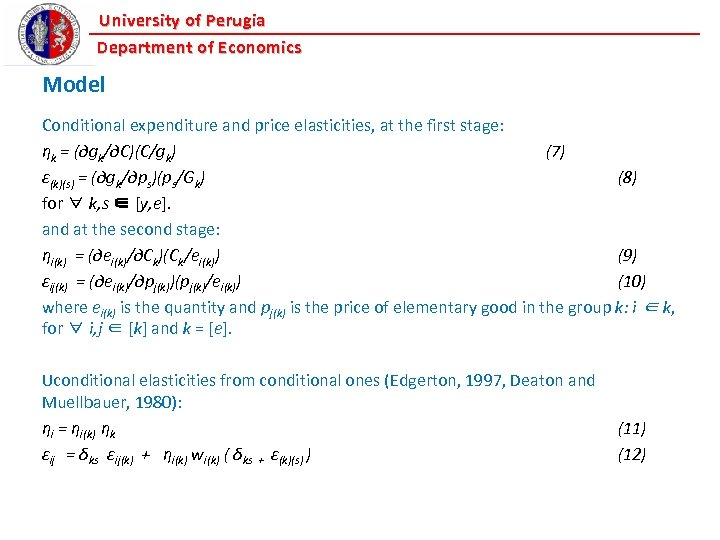University of Perugia Department of Economics Model Conditional expenditure and price elasticities, at the first stage: ηk = (∂gk/∂C)(C/gk) (7) ε(k)(s) = (∂gk/∂ps)(ps/Gk) (8) for ∀ k, s ∈ [y, e]. and at the second stage: ηi(k) = (∂ei(k)/∂Ck)(Ck/ei(k)) (9) εij(k) = (∂ei(k)/∂pj(k))(pj(k)/ei(k)) (10) where ei(k) is the quantity and pj(k) is the price of elementary good in the group k: i ∈ k, for ∀ i, j ∈ [k] and k = [e]. Uconditional elasticities from conditional ones (Edgerton, 1997, Deaton and Muellbauer, 1980): ηi = ηi(k) ηk εij = δks εij(k) + ηi(k) wi(k) ( δks + ε(k)(s) ) (11) (12)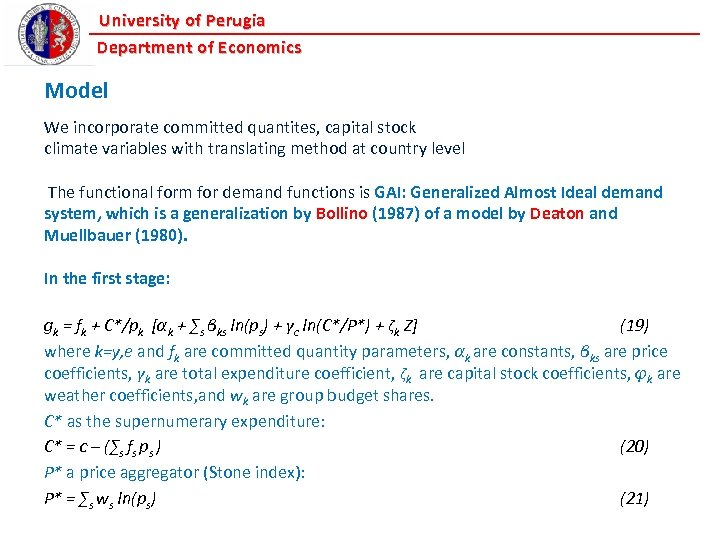University of Perugia Department of Economics Model We incorporate committed quantites, capital stock climate variables with translating method at country level The functional form for demand functions is GAI: Generalized Almost Ideal demand system, which is a generalization by Bollino (1987) of a model by Deaton and Muellbauer (1980). In the first stage: gk = fk + C*/pk [αk + ∑s βks ln(ps) + γc ln(C*/P*) + ζk Z] (19) where k=y, e and fk are committed quantity parameters, αk are constants, βks are price coefficients, γk are total expenditure coefficient, ζk are capital stock coefficients, φk are weather coefficients, and wk are group budget shares. C* as the supernumerary expenditure: C* = c – (∑s fs ps ) (20) P* a price aggregator (Stone index): P* = ∑s ws ln(ps) (21)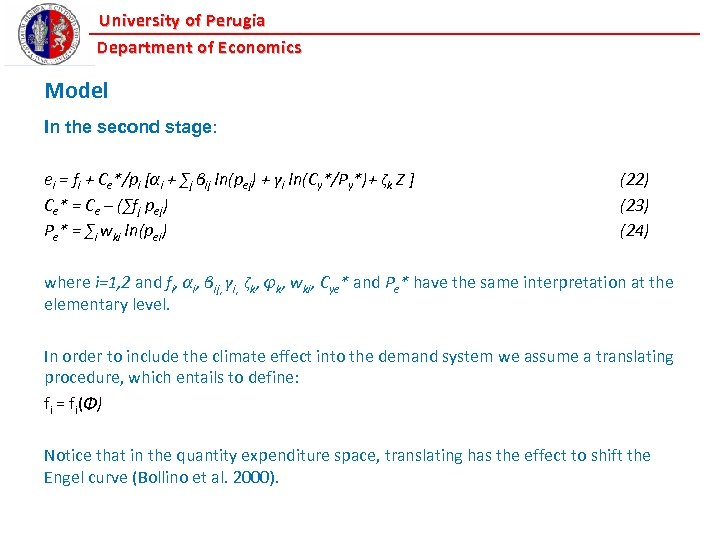University of Perugia Department of Economics Model In the second stage: ei = fi + Ce*/pi [αi + ∑j βij ln(pej) + γi ln(Cy*/Py*)+ ζk Z ] Ce* = Ce – (∑fj pej) Pe* = ∑i wki ln(pei) (22) (23) (24) where i=1, 2 and fi, αi, βij, γi, ζk, φk, wki, Cye* and Pe* have the same interpretation at the elementary level. In order to include the climate effect into the demand system we assume a translating procedure, which entails to define: fi = fi(Φ) Notice that in the quantity expenditure space, translating has the effect to shift the Engel curve (Bollino et al. 2000).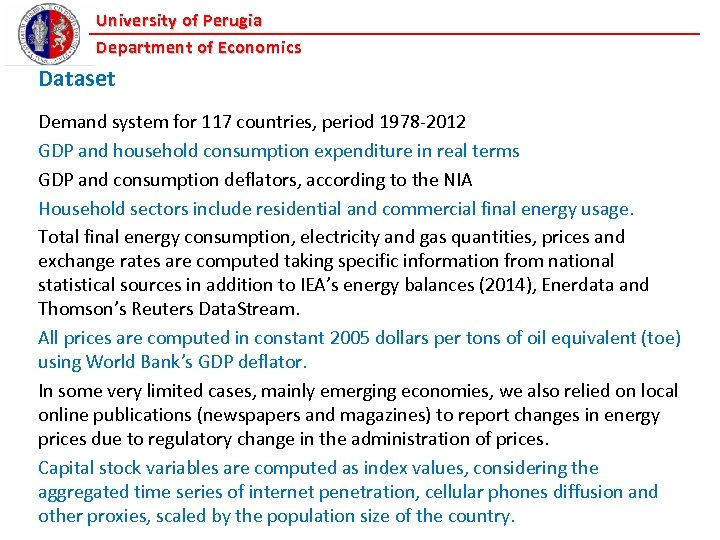University of Perugia Department of Economics Dataset Demand system for 117 countries, period 1978 -2012 GDP and household consumption expenditure in real terms GDP and consumption deflators, according to the NIA Household sectors include residential and commercial final energy usage. Total final energy consumption, electricity and gas quantities, prices and exchange rates are computed taking specific information from national statistical sources in addition to IEA’s energy balances (2014), Enerdata and Thomson’s Reuters Data. Stream. All prices are computed in constant 2005 dollars per tons of oil equivalent (toe) using World Bank’s GDP deflator. In some very limited cases, mainly emerging economies, we also relied on local online publications (newspapers and magazines) to report changes in energy prices due to regulatory change in the administration of prices. Capital stock variables are computed as index values, considering the aggregated time series of internet penetration, cellular phones diffusion and other proxies, scaled by the population size of the country.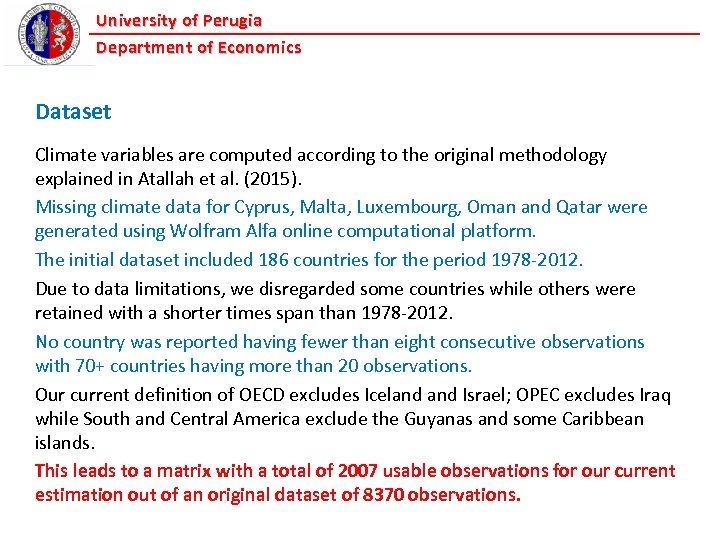University of Perugia Department of Economics Dataset Climate variables are computed according to the original methodology explained in Atallah et al. (2015). Missing climate data for Cyprus, Malta, Luxembourg, Oman and Qatar were generated using Wolfram Alfa online computational platform. The initial dataset included 186 countries for the period 1978 -2012. Due to data limitations, we disregarded some countries while others were retained with a shorter times span than 1978 -2012. No country was reported having fewer than eight consecutive observations with 70+ countries having more than 20 observations. Our current definition of OECD excludes Iceland Israel; OPEC excludes Iraq while South and Central America exclude the Guyanas and some Caribbean islands. This leads to a matrix with a total of 2007 usable observations for our current estimation out of an original dataset of 8370 observations.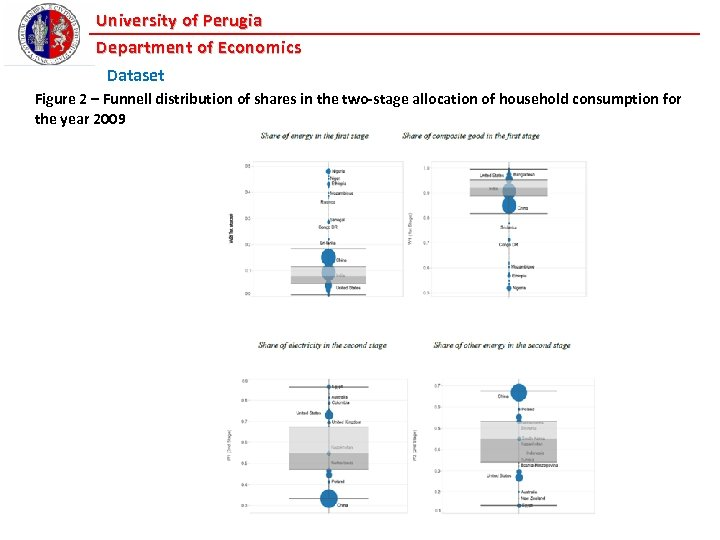University of Perugia Department of Economics Dataset Figure 2 – Funnell distribution of shares in the two-stage allocation of household consumption for the year 2009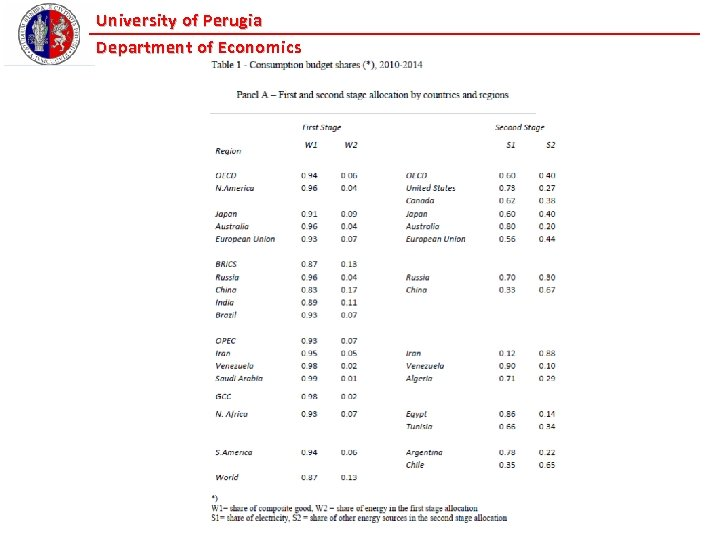University of Perugia Department of Economics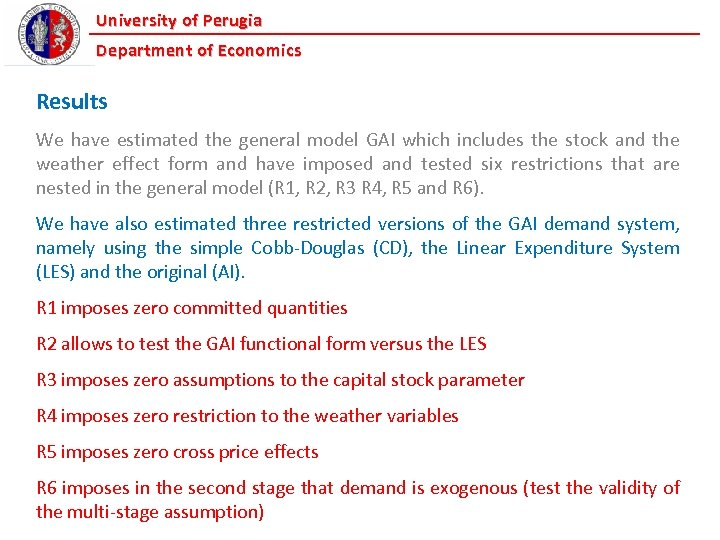University of Perugia Department of Economics Results We have estimated the general model GAI which includes the stock and the weather effect form and have imposed and tested six restrictions that are nested in the general model (R 1, R 2, R 3 R 4, R 5 and R 6). We have also estimated three restricted versions of the GAI demand system, namely using the simple Cobb-Douglas (CD), the Linear Expenditure System (LES) and the original (AI). R 1 imposes zero committed quantities R 2 allows to test the GAI functional form versus the LES R 3 imposes zero assumptions to the capital stock parameter R 4 imposes zero restriction to the weather variables R 5 imposes zero cross price effects R 6 imposes in the second stage that demand is exogenous (test the validity of the multi-stage assumption)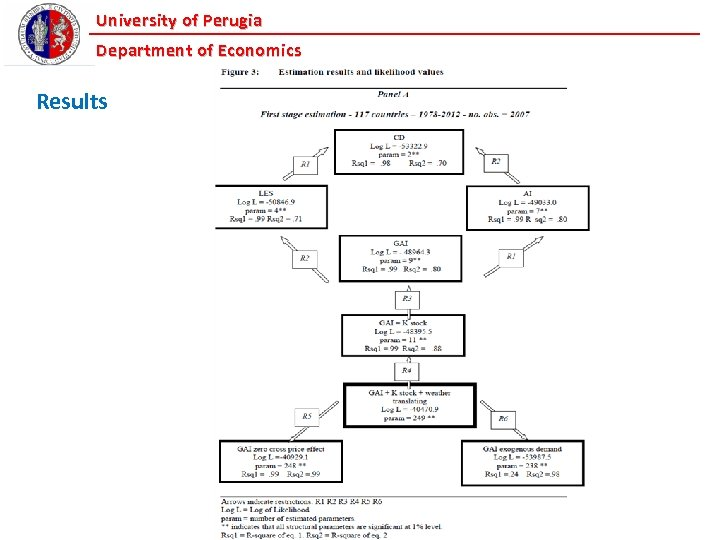University of Perugia Department of Economics Results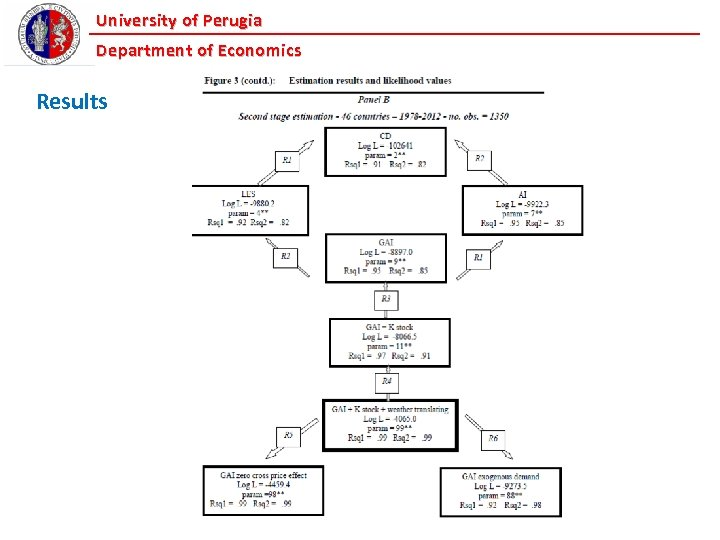University of Perugia Department of Economics Results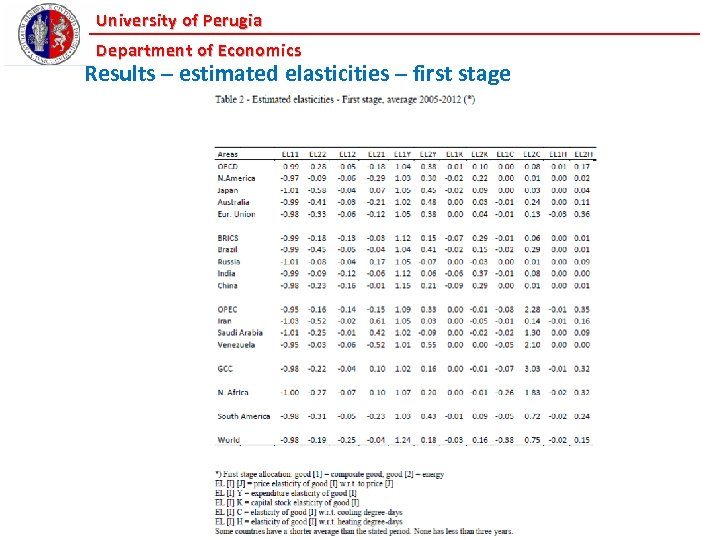University of Perugia Department of Economics Results – estimated elasticities – first stage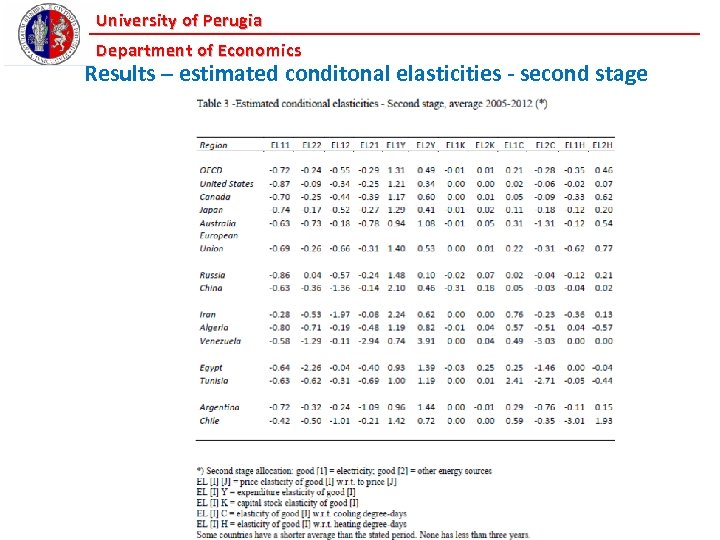University of Perugia Department of Economics Results – estimated conditonal elasticities - second stage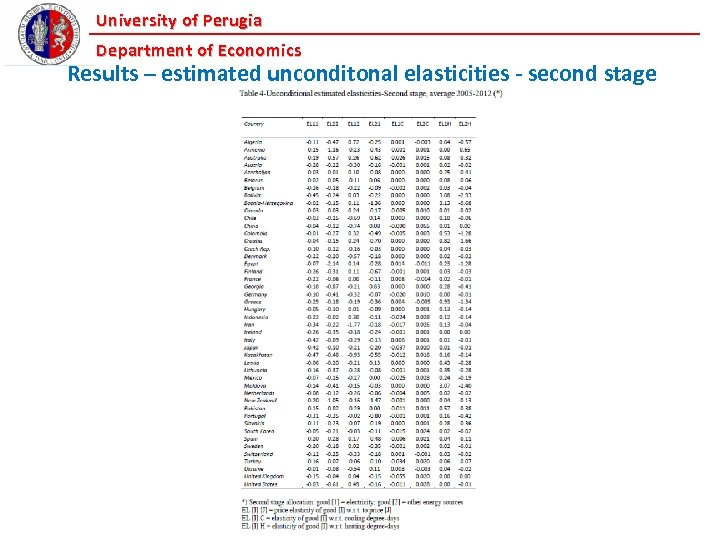University of Perugia Department of Economics Results – estimated unconditonal elasticities - second stage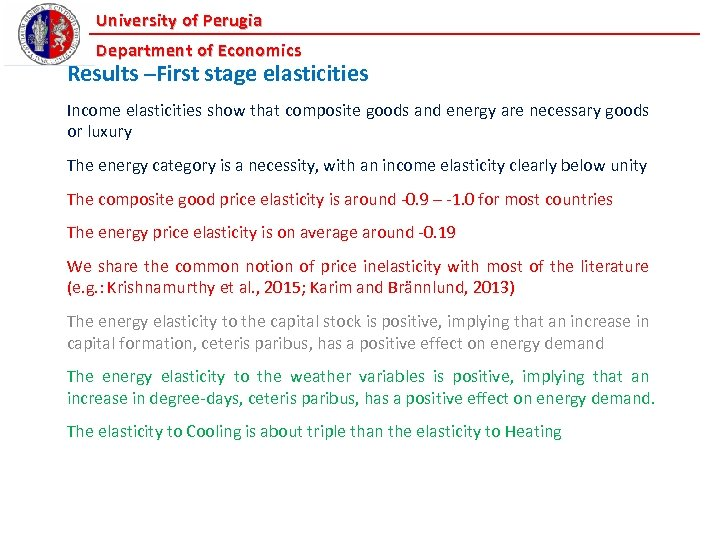University of Perugia Department of Economics Results –First stage elasticities Income elasticities show that composite goods and energy are necessary goods or luxury The energy category is a necessity, with an income elasticity clearly below unity The composite good price elasticity is around -0. 9 – -1. 0 for most countries The energy price elasticity is on average around -0. 19 We share the common notion of price inelasticity with most of the literature (e. g. : Krishnamurthy et al. , 2015; Karim and Brännlund, 2013) The energy elasticity to the capital stock is positive, implying that an increase in capital formation, ceteris paribus, has a positive effect on energy demand The energy elasticity to the weather variables is positive, implying that an increase in degree-days, ceteris paribus, has a positive effect on energy demand. The elasticity to Cooling is about triple than the elasticity to Heating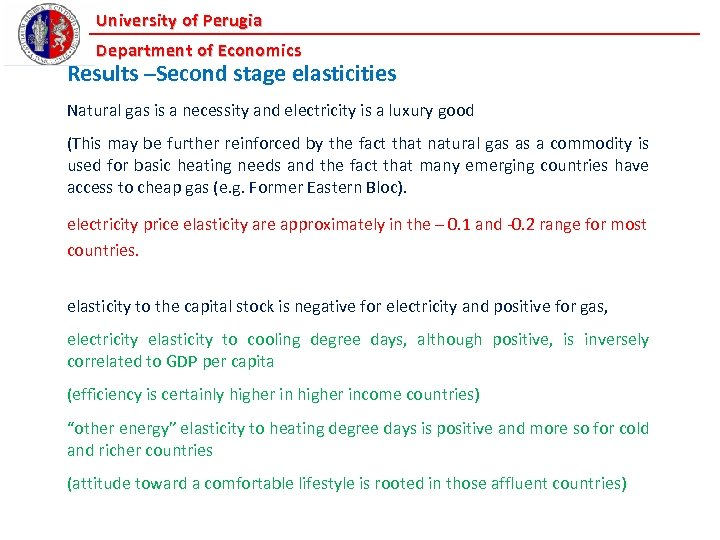University of Perugia Department of Economics Results –Second stage elasticities Natural gas is a necessity and electricity is a luxury good (This may be further reinforced by the fact that natural gas as a commodity is used for basic heating needs and the fact that many emerging countries have access to cheap gas (e. g. Former Eastern Bloc). electricity price elasticity are approximately in the – 0. 1 and -0. 2 range for most countries. elasticity to the capital stock is negative for electricity and positive for gas, electricity elasticity to cooling degree days, although positive, is inversely correlated to GDP per capita (efficiency is certainly higher income countries) “other energy” elasticity to heating degree days is positive and more so for cold and richer countries (attitude toward a comfortable lifestyle is rooted in those affluent countries)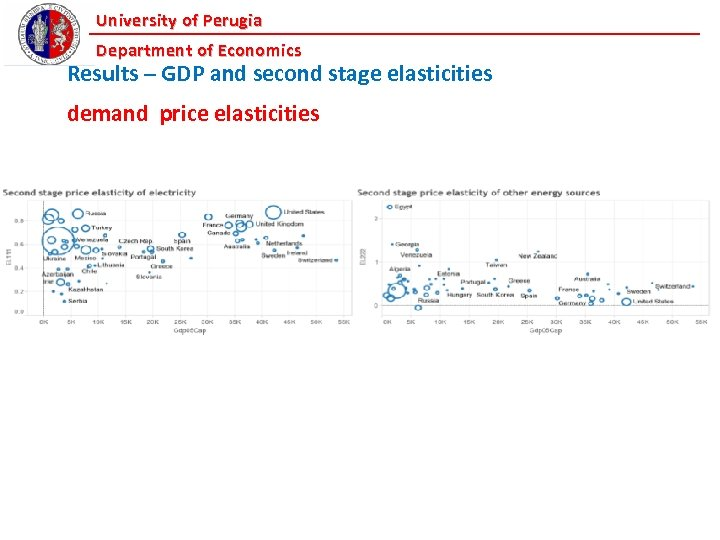University of Perugia Department of Economics Results – GDP and second stage elasticities demand price elasticities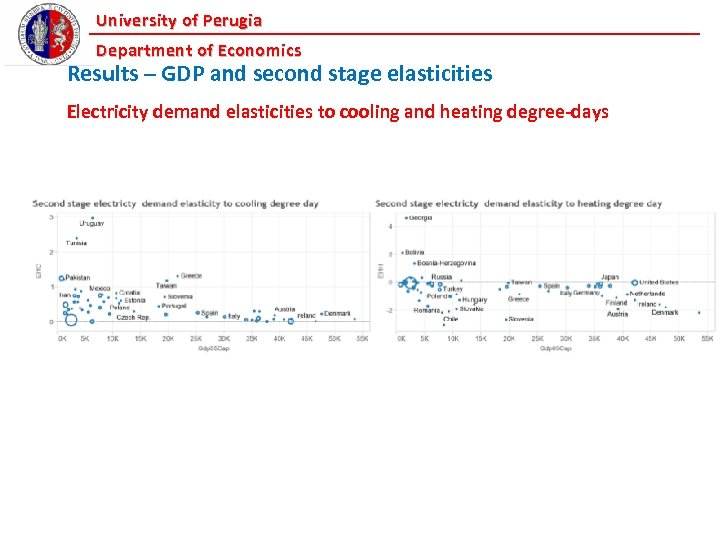University of Perugia Department of Economics Results – GDP and second stage elasticities Electricity demand elasticities to cooling and heating degree-days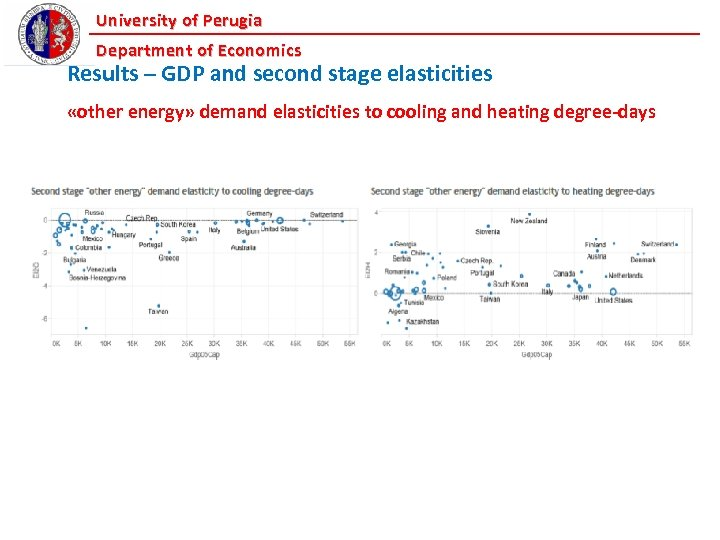University of Perugia Department of Economics Results – GDP and second stage elasticities «other energy» demand elasticities to cooling and heating degree-days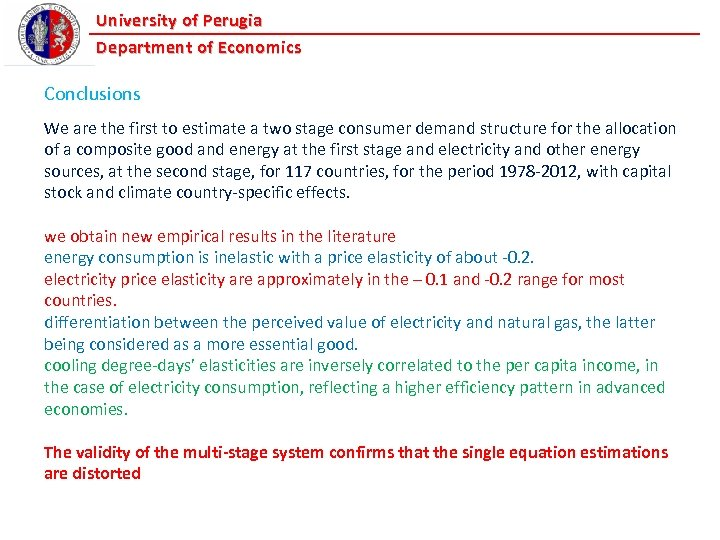University of Perugia Department of Economics Conclusions We are the first to estimate a two stage consumer demand structure for the allocation of a composite good and energy at the first stage and electricity and other energy sources, at the second stage, for 117 countries, for the period 1978 -2012, with capital stock and climate country-specific effects. we obtain new empirical results in the literature energy consumption is inelastic with a price elasticity of about -0. 2. electricity price elasticity are approximately in the – 0. 1 and -0. 2 range for most countries. differentiation between the perceived value of electricity and natural gas, the latter being considered as a more essential good. cooling degree-days’ elasticities are inversely correlated to the per capita income, in the case of electricity consumption, reflecting a higher efficiency pattern in advanced economies. The validity of the multi-stage system confirms that the single equation estimations are distorted